﻿ Real-Time Object Tracking by CUDA-accelerated Neural Network

### Real-Time Object Tracking by CUDA-accelerated Neural Network

Mikhail S. Tarkov, Sergey V. DubyninOPEN ACCESSPEER-REVIEWED

## Real-Time Object Tracking by CUDA-accelerated Neural Network

Mikhail S. Tarkov1,, Sergey V. Dubynin2

1A.V. Rzhanov's Institute of Semiconductor Physics SB RAS, Novosibirsk, Russia

2Novosibirsk State University, Novosibirsk, Russia

### Abstract

An algorithm is proposed for tracking objects in real time. The algorithm is based on neural network implemented on GPU. Investigation and parameter optimization of the algorithm are realized. Tracking process has accelerated by 10 times and the training process has accelerated by 2 times versus to the sequential algorithm version. The maximum resolution of the frame for real-time tracking and the optimum frame sampling from a movie are calculated.

### At a glance: Figures

12
Prev Next

• Tarkov, Mikhail S., and Sergey V. Dubynin. "Real-Time Object Tracking by CUDA-accelerated Neural Network." Journal of Computer Sciences and Applications 1.1 (2013): 1-4.
• Tarkov, M. S. , & Dubynin, S. V. (2013). Real-Time Object Tracking by CUDA-accelerated Neural Network. Journal of Computer Sciences and Applications, 1(1), 1-4.
• Tarkov, Mikhail S., and Sergey V. Dubynin. "Real-Time Object Tracking by CUDA-accelerated Neural Network." Journal of Computer Sciences and Applications 1, no. 1 (2013): 1-4.

 Import into BibTeX Import into EndNote Import into RefMan Import into RefWorks

### 1. Introduction

Currently, the distribution and development of video takes enormous size. One of the most common problems in this field is the object tracking. The object tracking algorithms are used for various purposes: identification of specific moving targets, fixing car license plates, imposition of various visual effects to the video etc.

To implement tracking objects, many methods and algorithms are developed but often they are highly specialized and are stable only in a certain type of video. In good conditions (clear images at a low speed of the object), these algorithms work well but in case of noise, increasing the speed of the object and reducing its size, the algorithms glitch. On top of the object tracking algorithms are quite labor intensive and forcing compress the processed image or otherwise simplify processed information. In this regard, there is a problem to develop effective algorithms for robust object tracking.

The objective of this work is to develop a parallel algorithm, and to apply a graphic accelerator to speed up the image processing without reducing its size.

We obtain the following results:

1. The sequential neural network object-tracking algorithm [1, 2] is investigated, and its parallel version is developed using the CUDA technology .

2. A comparison of serial and parallel algorithms is realized on several parameters: the speed of object tracking and the neural network training, and the maximum size of the frame acceptable for real-time processing. The parallel algorithm speedup is more than 10.

### 2. Problem Statement

There are many different systems for tracking objects. These systems use different algorithms and operate with different input data. The most effective implementations use complex and expensive equipment: multiple cameras and record color video or video in the infrared spectrum. On the one hand, the color image allows us to use many different algorithms, but on the other hand, these algorithms can be time-consuming and may not always work properly (for example, in low-light) . Algorithms for monochrome images can use a cheaper technology, but are less effective and often use low-resolution frame, which is caused by the specific equipment .

The algorithm  is a fairly simple algorithm that works with monochrome images of low resolution (320x240), which during the pre-processing of data is reduced to 80x60. However, we can create a fast algorithm that works with a large frame resolution in real-time on a fairly low-cost hardware. The key to this solution lies in the use of graphics cards as devices that perform massively parallel computing .

The overall objective is to track the object in images and video that is to determine the coordinates of the center of the object on the basis of information obtained from the image. Since one of the goals is to observe the object in the video in real time, then the algorithm for determining the coordinates of the center of the object is superimposed on speed limitation: the processing of a single frame should take no more than 1/25 sec. = 0.04 sec. Another aim is to develop an algorithm that allows to process the entire frame, with no loss of information. At the same time, in  every fourth pixel of the image is used only to increase the speed of the algorithm.

### 3. Neural Network and Its Training

We used sigmoid feedforward network with one hidden layer [1, 2]. Before processing the normalization of brightness of monochrome images in the range (0, 1) is performed.

The function of the neural network can be divided into three parts:

1) Input vector x is multiplied by the weight matrix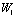of the hidden layer and then added to the displacement vector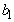:(1)

2) The activation function f is applied to the vector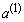: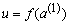(2)

3) The resulting vector u is multiplied by the weight matrixof the output layer and then added to the displacement vector: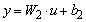(3)

As a result of the study of behavior of network with different numbers of neurons in the hidden layer, we decide to use the 64 neurons that provide sufficient speed and accuracy of the algorithm. In the output layer there are only two neurons, each of which should give on the output one of the coordinates of the desired object.

To train the neural network a set of images with known coordinates of the center of the object is used. As training algorithm the backpropagation algorithm  was chosen.

Minimized the objective function of the neural network error is the quantity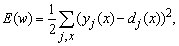(4)

where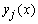is the real output state of the neuron j of the output layer of the neural network when the input imageis applied,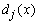is the desired output state of the neuron.

Minimization is the method of gradient descent:(5)

whereis the change of the component of the matrix of weights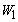() or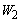(),. The initial values of weights are set randomly.

Training by backpropagation is in accordance with the following paragraphs:

1. Select the first input vector of the training set.

2. Supply the selected vector to the input of the neural network and calculate its output.

3. Calculate the error for the output layer: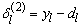(6)

4. Calculate the change of weights for the output layer:(7)

5. Calculate the error for the hidden layer: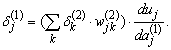(8)

6. Calculate the change of weights for the hidden layer: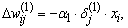(9)

whereis the component of the input vector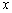.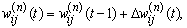(10)

where t is the number of iteration of the training process.

8. If the training set has untreated vectors, select a vector and go to step 2.

9. Find the total error on the test set by the formula (4). If the error for the previous image is more than the error for the current image, increase values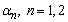, else decrease them. If the error is less than the previous record, change record:(11)

Save the values of the weights(12)

and go to step 1.

If the record has not been changed for a certain number of passes of steps 1-8, complete the training and restore the most successful weight record.

Training ratesand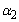are adjusted by using the parameters,and. All these parameters are close to 1. For example,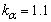, .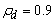.,. If(the error is increased), thenandare multiplied by(decreased), else they are multiplied by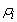(increased). The parameters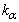,иare determined experimentally.

To create a set of images the program Autodesk Maya 2011  was used, which created a three-dimensional model of the gear (Figure 1).

For this model, using formulas depending on the frame number, the following parameters are evaluated: the coordinates of the object in the frame, the angle of rotation of the object in three axes and the object size. Then the object video was obtained for testing the object tracking. A program was realized for describing the object movement and recording the coordinates of the center of the object to the file.

To implement the parallel algorithm, the CUDA  hardware and software architecture is used. It performs calculations using graphics processors NVIDIA enabled for GPGPU (general purpose computations on graphics cards). For preliminary processing and output of information in the course of the neural network training the computer vision library OpenCV  is used.

### 4. CUDA Implementation

CUDA (Compute Unified Device Architecture) is a framework which allows to develop C/C++ programs that execute specific functions (so-called kernels) on a CUDA-compatible graphics card in parallel. This graphics card is called device in this context. The computer on which the device is installed is called host. An instance of a kernel is called a grid. It consists of an arbitrary number of blocks. Each block consists of the same number of threads, which all execute the kernel’s code in parallel.

During the execution of a grid, blocks and threads are mapped to the multiprocessors of the GPU (Graphics Processing Unit) and their (scalar) processors, respectively.

A kernel may use multiple kinds of memory: registers, shared memory, texture cache and constant cache are fast, but small on-chip memory, whereas device memory is much larger (up to 2GB), but has a drastically higher latency. Registers are accessible only from the current thread, shared memory is accessible from all threads of one block. Data transfer between blocks as well as between the host and the device can only be accomplished via the device memory.

All neurons of one layer perform calculations independently. In this regard, we decided to implement parallel versions of the neural network main functions and its weight training procedures.

We have three functions containing a large number of operations that can be performed in parallel. These functions are implemented as core GPU functions running in parallel on multiple threads.

The most noteworthy is the kernel function (1). Similarly running kernel function (3). The function (2) is very simple, and its GPU realization does not require optimization.

In the preliminary version of the function (1) implementation, each thread performs the multiplication of one row of the matrixby the vector x. The number of threads corresponds to the number of neurons in the hidden layer. Each thread performs the operations of addition and multiplication, but the global memory GPU greatly slowing the threads.

Besides the Computer Visual Profiler shows that we are not using the combining of requests to the memory: when successive threads turn into consecutive memory locations, the treatment can be combined into one warp and instead group of calls we have in fact only one call. The maximum number of threads within the warp is 16 or 32.

In the end, we decided to introduce a number of changes, which can eliminate these disadvantages:

1) Increase the number of threads so that each thread only multiplies the vector of 16 numbers. This increases the payload on the GPU, more threads run in parallel, there is a need for a modified kernel function, summarizing the results of the threads.

2) Use shared memory instead of global one. This memory is allocated to each block of threads and can be used by all threads of the block . The idea is to get a fragment of global memory used by all the threads of the block in shared memory. Each thread makes only one reference to the corresponding cell of global memory by copying the value in shared memory, and the other data the thread will be able to get from the shared memory.

3) Transpose matrixto access elements of the matrix in global memory by warp. Initially every thread worked with a row vector as with consecutive memory elements. Thus, the threads, going after each other for numbering, refer to different segments of memory. Matrix transposition allows the threads to work with a column vector that combines consecutive threads in warp.

As a result of these modifications, the total execution time for the kernel function of the first block on the program run decreased from 30% of the GPU time to 1% plus 3% to the kernel accumulating function appeared in the course of the change 1. All uploads from the global memory and downloads to the global GPU memory are coalesced. The function loop branching and the warp starting took very little time.

In the functions of adjusting weights, operations (steps 4-7) are organized into kernel functions that run in parallel on multiple threads. However, the specifics of these blocks does not allow them a lot faster and forces to use matrix transposition before calling the function tuning the weights and after that, because the next function iteration must have transposed matrix of weights.

### 5. Experiments

The algorithm has a number of options that are determined experimentally. One such parameter is the number of neurons in the hidden layer. Due to the nature of parallel implementation of the algorithm we consider the number of neurons multiples of 16 (the size of a warp equals 16). As a result, it is observed that 16 and 32 neurons cannot provide the storing patterns. The 48 neurons cope with learning only small training set. The 64 neurons provided a good degree of storing patterns and satisfactory training speed. Further increases in the number of neurons leads only to reduce the speed of the neural network’s training and functioning.

In addition to the above parameters, attention also deserves the frequency with which you want to take pictures from the video in order to effectively train a neural network.

To train the neural network a sequence of 200 images with a resolution 80x60, 160x120, 320x240, 640x480, 800x600, and 1280x960 was used. A testing sequence has also 200 images. Figure 2 shows that even at a frequency of taking one frame out of ten, a satisfactory result is achieved (sum of maximum deviations in both coordinates is 50 pixels when the object size is 300x300 in frame with size 1280x960). Further increase of the frame rate only slows the learning process, not giving a significant gain in quality. Number of training cycles (epochs) was about 2200.

Testing the parallel and serial implementations were carried out on a computer with the following characteristics: CPU - AMD Athlon 7750, 2 cores at 2.7 GHz, GPU - NVIDIA GeForce 9800 GT with 512MB memory, the number of thread processors is 112. Development of a parallel version was conducted using Cuda Toolkit 4.1.

The main investigated parameter is the speed of the neural network that is the main function of object tracking.

From Figure 3 it follows that the parallel implementation of the neural network on GPU can increase the linear dimensions of the processed image by 4 times (from 320240 to 1280960). From Figure 3 and Figure 4 it follows that the processing of frame sequence by the neural network is accelerated by an average of 10. The training process is accelerated by an average of only 2 (Figure 5). This is due to the need to transpose the weight matrix in the implementation of training a neural network on the GPU.

### 6. Conclusion

An algorithm of tracking objects in real time, based on neural network learning algorithm with back propagation, is implemented in parallel on GPU. Investigation and parameter optimization of the algorithm are realized. Tracking process has accelerated by 10 times and the training process has accelerated by 2 times versus to the sequential algorithm version. The maximum resolution of the frame, suitable for real-time tracking, and the optimum frequency of capture frames from a movie in the training set are calculated.

The obtained algorithm acceleration is not the possible maximum, so further development in this area can give better results, both in performance on the previous frame resolution and the ability to handle a greater volume of information. It may be possible to develop algorithms training the neural network in real time, i.e. in a process of the object tracking.

### References

  Ahmed, J., Jafri, M. N., Ahmad, J., and Khan, M. I., “Design and Implementation of a Neural Network for Real-Time Object Tracking,” Engineering and Technology, 6. 209-212. 2005.In article  Haykin, S. Neural Networks. A Comprehensive Foundation, Prentice Hall Inc., 1999.In article  David, B. Kirk, and Wen-mei, W. Hwu, Programming Massively Parallel Processors. A Hands-on Approach, NVIDIA Corporation, 2010.In article  Mobileye Advanced Driver Assistant System. URL: http://www.mobileye.comIn article  Automatic vehicle driving. URL: http://www.argo.ce.unipr.it/ARGO/english/In article  Maya – 3D Animation – Autodesk. URL: http://usa.autodesk.com/maya/In article  Bradski, G., Kaehler, A., Learning OpenCV, O’Reilly Media, Inc., USA, 2008.In article